# QUIZ PRACTICE Trigonometric Angles Graphing Sine and Cosine

• Slides: 16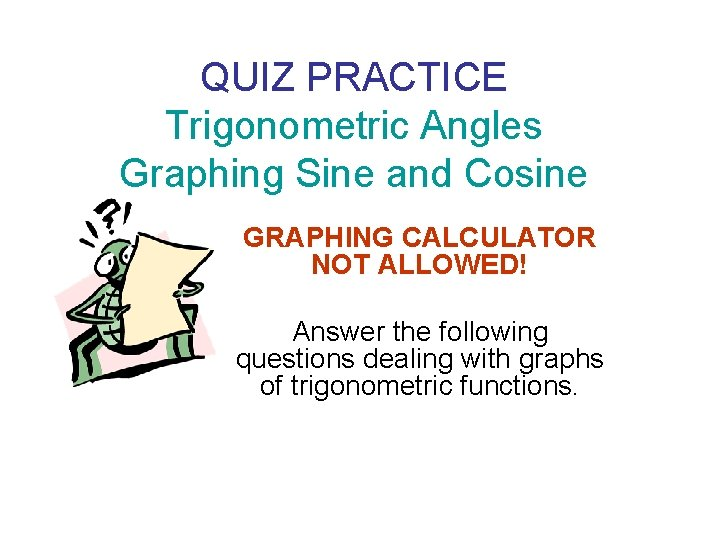QUIZ PRACTICE Trigonometric Angles Graphing Sine and Cosine GRAPHING CALCULATOR NOT ALLOWED! Answer the following questions dealing with graphs of trigonometric functions.1. Determine an equation for this graph:1. Determine an equation for this graph:2. Determine an equation for this graph: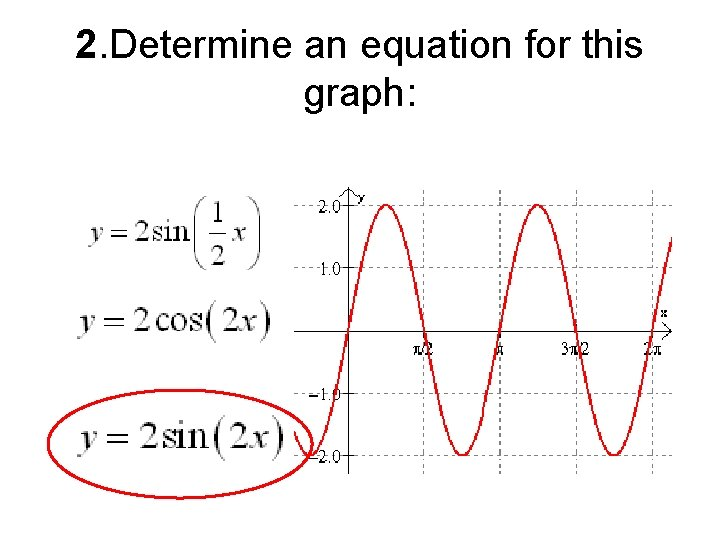2. Determine an equation for this graph: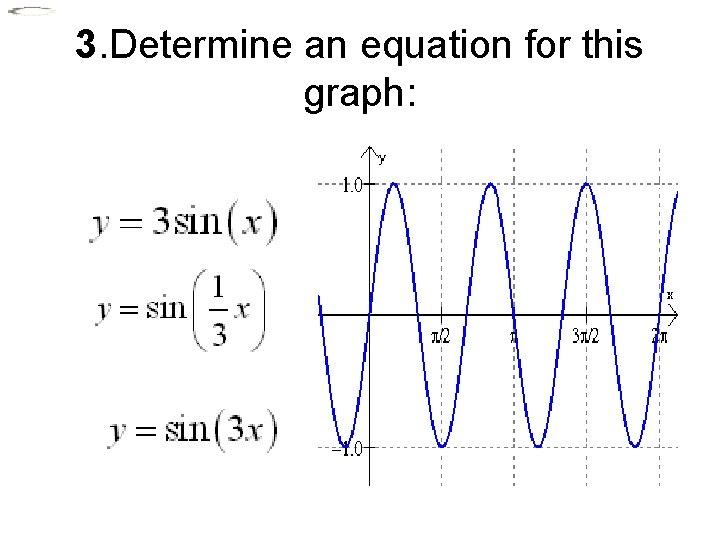3. Determine an equation for this graph:3. Determine an equation for this graph: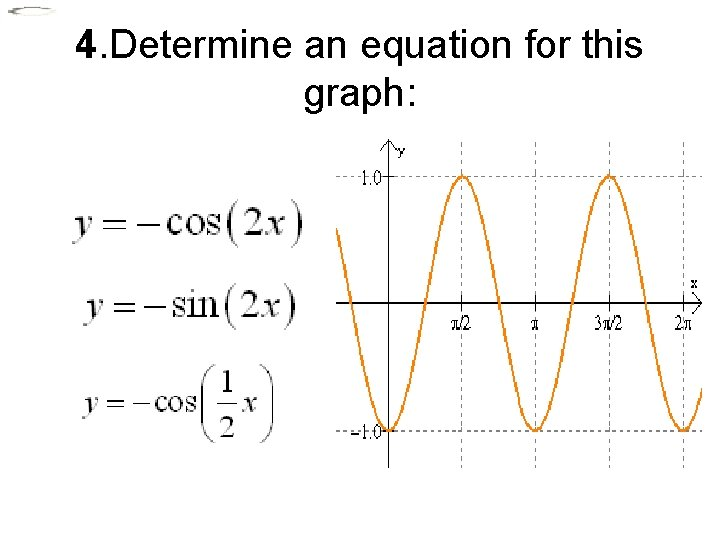4. Determine an equation for this graph: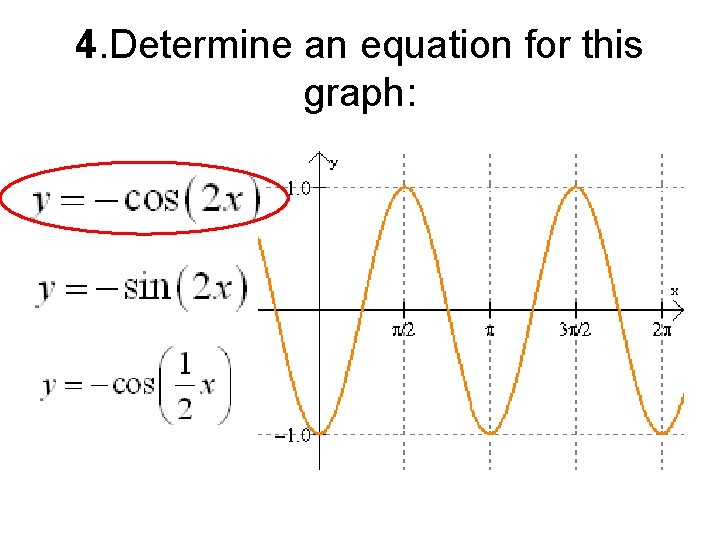4. Determine an equation for this graph: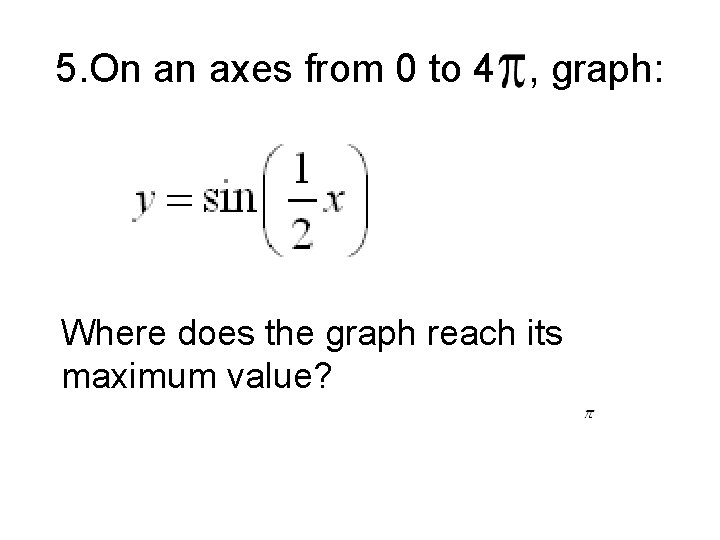5. On an axes from 0 to 4 , graph: Where does the graph reach its maximum value?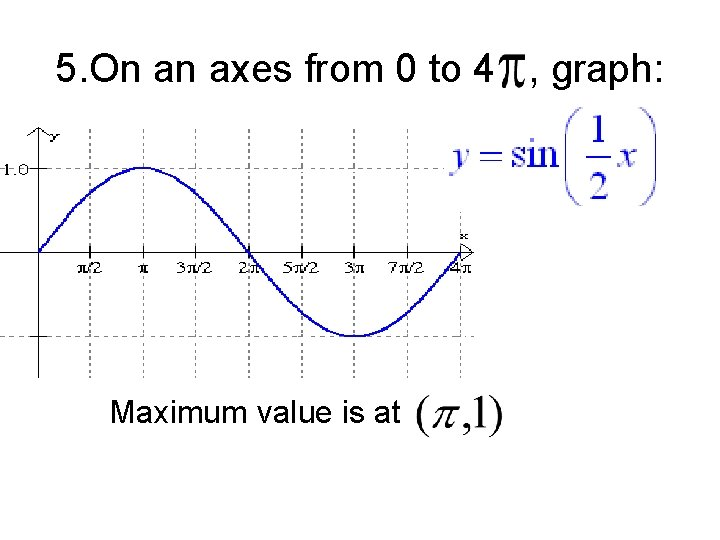5. On an axes from 0 to 4 , graph: Maximum value is at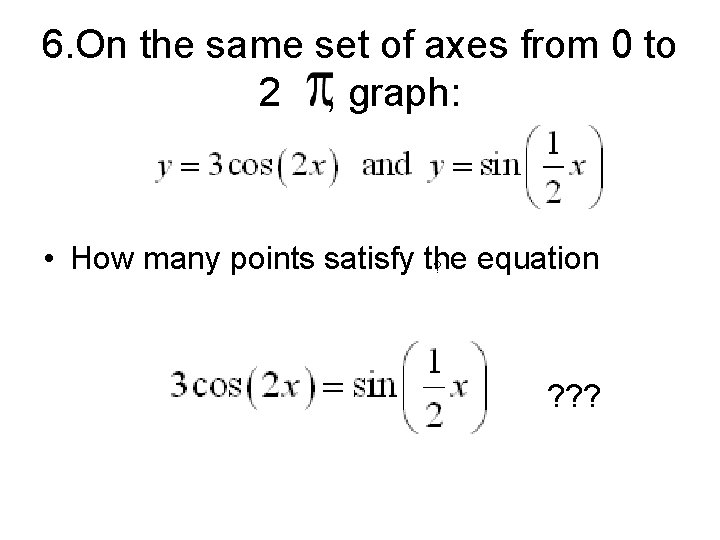6. On the same set of axes from 0 to 2 , graph: • How many points satisfy the equation ? ? ? ?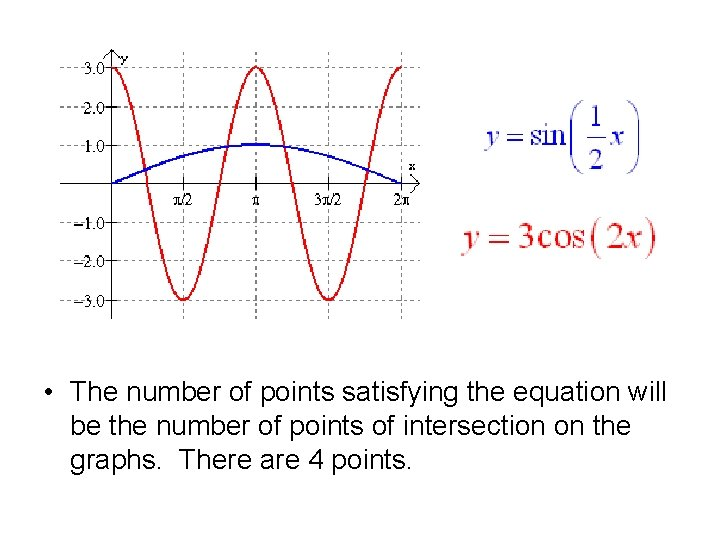• The number of points satisfying the equation will be the number of points of intersection on the graphs. There are 4 points.7. Write one sine and one cosine equation for the graph below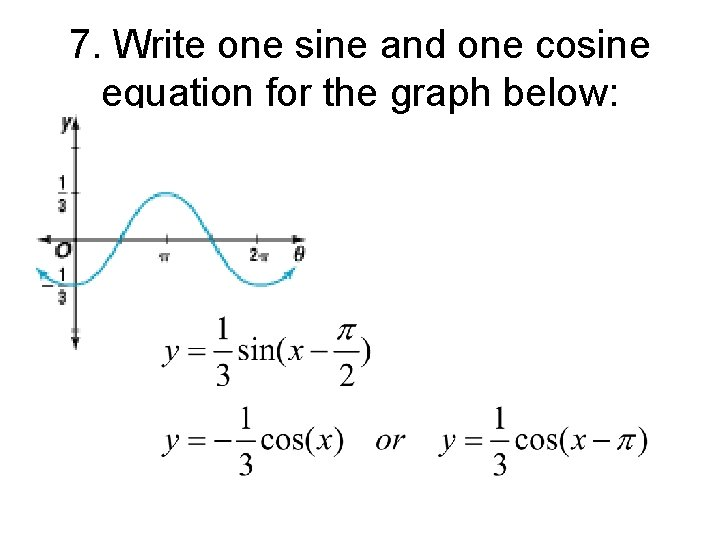7. Write one sine and one cosine equation for the graph below: